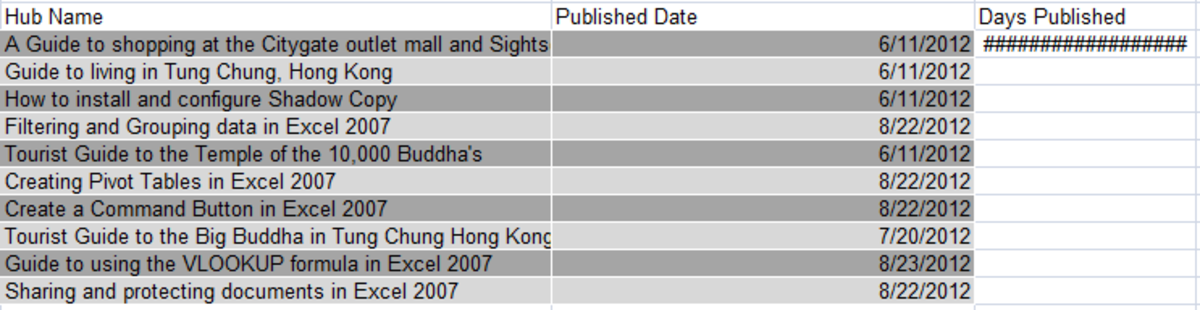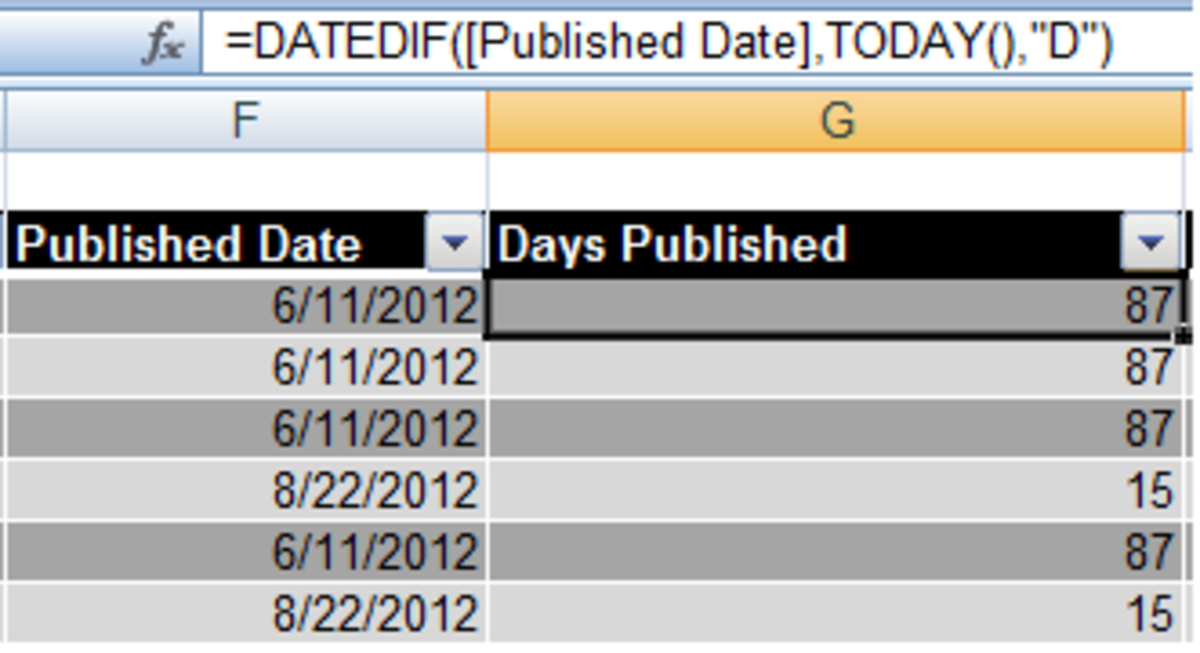# Using the DATEDIF, NETWORKDAYS, MONTH, YEAR and TODAY() functions in Excel 2007

## Introduction

Welcome to my latest hub on Excel 2007. Today I will be investigating using dates in Excel 2007. Specifically, I will be showing how to get Excel to display today’s date in a cell and also to calculate the duration in days, months or years between two dates using a number of different functions.

This hub came about when I thought it would be cool if I could get Excel 2007 to calculate the number of hits my hubs receive per day. I had three variables I needed to obtain to calculate this, the total number of hits, today’s date and the number of days my hubs have been published. I only had one of those variables to hand which is the total number of hits. So I set about discovering how Excel could help me with this problem. I began with learning about the function TODAY ().

## Using TODAY() in Excel 2007

The TODAY() function can be used in two ways. First, it can be used on its own to display the current date in a cell in Excel. It can also be used in formulas for date calculations. It is a very simple function, it has no arguments and can only be used as TODAY().

NOTE: When using the function in a formula, Excel stores the result as a date by default. To change this to a number, right click on the cell and select Format Cells and change Category to Number.

To get Excel to display today’s date in a cell, simply type in =TODAY() and press return.

## Using TODAY() in a formula

If you need to know the number of days since something has occurred, for example the number of days since your birthday, or in my example the number of days a hub has been published, you should use the TODAY() function. The syntax is very straight forward as you can see below.

Once you press enter, you will notice that the result is a series of #### as below. This is due to the formatting being set to Date. Change it to Number as outlined above and the correct result is displayed.The results of using TODAY() in a formula will display as ##### until formated as a number in Excel 2007.http://robbiecwilson.hubpages.com/

## Using the DATEDIF function in Excel 2007

While using TODAY() is perfect if you just want to know how many days have passed since a specific event, DATEDIF allows you to calculate using months and also years. To use the DATEIF formula in Excel 2007, you have to use the following syntax

=DATEDIF(<Start Date>,<End Date>,<Days, complete months or complete years (expressed as “D”,”M” or “Y”)>

The final argument can also contain combinations of years, days and months. For example,

MD – Excel returns the number of days between two dates while assuming that the dates were in the same month and year.

YD - Excel returns the number of days between two dates while assuming that the dates were in the same year.

YM - Excel returns the number of months between two dates while assuming that the dates were in the same year.

An example of the formula in use is shown below

As previously mentioned, ensure that the cell containing your formula is formatted as a number rather than a date so that the cell’s contents display correctly.

NOTE: The final argument, M, D or Y (or one of the other combinations) must be in speech marks “M”, “D” or “Y” or Excel 2007 will return an error.

Other useful date related Excel 2007 functions

Scroll to Continue

There are a number of other functions that are similarly useful when working with dates in Excel 2007.

## NETWORKDAYS

The first of these is NETWORKDAYS. This will calculate the number of working or business days between two dates. There is also an option of including a list of holidays in a range that Excel 2007 will subtract from the number of workdays. An example of the syntax is below

=NETWORKDAYS(G47,TODAY())

If I add some holidays as you can see below, I add in a final argument to include the range of holidays.

You can see that Excel gives the total as 78 when including my (made up) holidays and 80 without.

## MONTH

Another way of working out the number of months that have passed between two dates is to use the MONTH function. So I want to work out the number of months between the 6/11/2012 and today (note that the months are in the same year, if they are not use the formula in the next section). The formula I use for this is below.

## Formula for dates in different years

If you are calculating the number of months that have passed between two dates that are in a different year, the formula changes. You add in the following to the front of the formula

=(YEAR(TODAY())-YEAR(G47))*12+

Which becomes the following formula

=(YEAR(TODAY())-YEAR(G47))*12+MONTH(TODAY())-MONTH(G47)

NOTE: Again, note that YEAR occurs twice, just as MONTH has to.

NOTE: When working with this formula ensure that you use MONTH before your second date to ensure you don’t get a strange answer. If I leave out that second MONTH, Excel helpfully gives me the answer -41062 rather than the correct answer which is of course 3.

## YEAR

To determine the number of years between two dates, use the YEAR function as we did for determining the number of months between dates in different years.

=YEAR(<End Date>)-YEAR(<Start Date>)

## A Real life example of using dates in formulas

So, to finish off this hub on using dates, back to the original reason for my researching this in the first place. I wanted to calculate the number of hits per day that my hubs generate. To calculate the number of days since a hub was published, I used the following formulaAn example of the DATEDIF and TODAY() functions in a formula in Excel 2007.http://robbiecwilson.hubpages.com/

Now I have all the variables I need, so I simply divide the total number of hits by the number of days published and voila!

## Conclusion

Excel 2007 has a number of very useful functions for determining the number of days, years or months that have passed between two dates. You have the MONTH and YEAR function which simply calculate months or years, as well as the more powerful DATEIF which allows you more flexibility. The NETWORKDAYS function allows you to calculate the number of working days between two dates and even allows you to adjust for holidays. Finally, the TODAY() function allows you to use today’s date in formulas.

I do hope that you have enjoyed reading this hub as much as I enjoyed writing it. Please feel free to leave any comments you may have below.

I also have a number of other hubs on aspects of Excel 2007, covering everything from Conditional Formatting to creating charts and graphs. I have an Index hub which also covers how I successfully transitioned from Excel 2003 to 2007 as well as outlining my other Excel 2007 hubs which can be found here